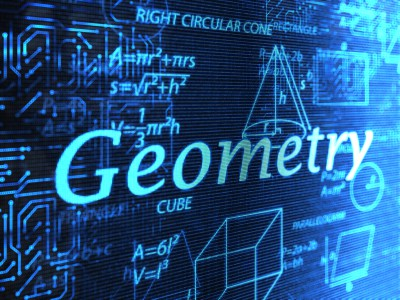# Quant Quiz on “GEOMETRY” for SSC CGL 2016

For the first time SSC 2016 examination will be held in Computer Based pattern and managing time will be one of the important factor while solving the questions. So in order to make students familiar with such a situations we are providing questions in a time based manner , which will help students to manage the time properly.1. If tan2B= (1 –sin⁡A)/(1 +sin A)then, find the value of A + 2B?

π/2
3π/2
5π/2
π

2. Find the value of (1+tan⁡75°)/(1 –tan⁡75° )?

-√3
2√3
0
1
Solution:

(1+tan⁡75°)/(1 –tan⁡75° )=(tan⁡45°+tan 75°)/(1–tan⁡45°.tan⁡75° )
= tan (45° + 75°) = tan 120°
= tan (180° – 60°) = – tan 60° = –√3

3. If Q is an acute angle and cos2q + cos4q = 1 then find the value tan2q + tan4q?

0
1
-1
None of the above

4. If cos4q – sin4q = 2/3, then find the value of 2 cos2q – 1?

0
2/3
4/3
1

5.

a
b
c
d

6. The value of (sec q – cos q)(cosec q – sin q) (tan q + cot q) is

1
2/3
2
0

7. If cot A + cosec A = 3 and A is an acute angle, then the value of cos A is :

4/5
2
1/2
3/4

8. Sin (45 + q) – cos (45 – q) is

2sinq
1
2 cosq
0

9. The least value of 2sin2q + 3cos2q is

3
5
1
2

10.

a
b
c
d

×

## Download success!

Thanks for downloading the guide. For similar guides, free study material, quizzes, videos and job alerts you can download the Adda247 app from play store.

Thank You, Your details have been submitted we will get back to you.

## Leave a comment

×
Login
OR

Forgot Password?

×
Sign Up
ORForgot Password
Enter the email address associated with your account, and we'll email you an OTP to verify it's you.Reset Password
Please enter the OTP sent to
/6

×
CHANGE PASSWORD